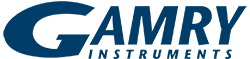# Rotational Speed of Rotating Disk Electrodes

### Introduction

Since the mid-20th century, rotating disk electrodes (RDEs) have been used to provide a steady stream of reactants “uniformly accessible” to the surface of a disk. The RDE was first examined theoretically by Veniamin Levich in the early 1950s. In an RDE, the bulk solution around the electrode may be roughly divided into two volumes: the convection-stirred solution away from the electrode; and the stagnant hydrodynamic boundary layer immediately next to the disk electrode, carried along with the disk’s rotation. As a redox reaction occurs at the RDE, products leave the boundary layer, and reactants enter the boundary layer from the bulk solution.

The thickness of the hydrodynamic boundary layer (δH) can be approximated as

### δH = 3.6(v/ω)1/2

in terms of the kinematic viscosity of the solution (v) and the angular rotation rate (ω = 2πf / 60, where f is the rotation rate in revolutions per minute). In an aqueous solution at a moderate rotation rate (~1000 rpm), the stagnant layer is approximately 300 to 400 µm thick.

How important is high rotational speed of the electrode for your experiment, though?

The Levich Equation

Levich provided a mathematical treatment of the convection and diffusion near an RDE. For a simple case, let us consider when only the oxidized form of a molecule (or ion) of interest is initially present in the electrochemical cell. The cathodic limiting current (iLC) observed at an RDE is given by the Levich Equation,

where CO is the concentration of the oxidized form in the solution, F is the Faraday constant (F = 96485 C mol–1),A is area of the electrode,v is the kinematic viscosity of the

solution, D is the diffusion coefficient of the oxidized, and ω is the angular rotation rate given above.

When the solution initially contains only the reduced form, the Levich Equation for the anodic limiting current (iLA) can be written as

where CR stands for the reduced form rather than the oxidized form.

Levich Study

Most researchers use the Levich Equation in Levich studies performed using an RDE, in which a series of voltammograms is acquired over a range of rotation rates. When a large-enough voltage is applied during a voltammogram, you measure the rate of reaction that is governed only by mass-transport to the electrode surface. The current measured here is the diffusion-limited current, or simply limiting current. For a simple electrochemical system where the rate of the half reaction is governed only by mass transport to the electrode surface, the limiting current should increase with the square root of the rotation rate.

The currents measured during a Levich study are usually plotted against the square root of the rotation rate on a graph called a Levich plot (see Fig. 1). As predicted by the Levich Equation, the limiting current increases linearly with the square root of the rotation rate (with a slope of 0.620 nFAD2/3v1/6C) and the line intercepts the vertical axis at zero. Often experimenters choose a set of rotation rates that are multiples of perfect squares (e.g., 400, 900, 1600 rpm, etc.) to make constructing the plot easier.Figure 1. Levich study, limiting current vs square root of the rotation rate.

The current, given the Levich Equation, is a limiting current, at the theoretical speed of 0 rpm, where the system behaves like a standard non-rotating electrode.

Koutecký-Levich Analysis

Another way to analyze the data from a Levich study is to plot the reciprocal of the limiting current:This is called a Koutecký-Levich plot (see Fig. 2), and is applicable to a system with a simple and reversible half-reaction. In this plot the data lie on a straight line with a vertical intercept at zero. If the line intercepts the vertical axis above zero this indicates that the half-reaction is limited by sluggish kinetics rather than by mass transport. The y-intercept itself is the inverse of the kinetic current iK. Finding the kinetic current at a variety of different overpotentials along the voltammogram can give you the standard rate constant for the half-reaction.Figure 2. Koutecký-Levich study, inverse limiting current vs inverse square root of the rotation rate.

Rotational Speed in a Koutecký-Levich Analysis

The x-axis of a Koutecký-Levich plot is the inverse of the square-root of the rotational rate ω. Thus the faster the disk electrode spins, the asymptotically closer to zero the data get. You can see that in such a plot, beyond a certain practical rotational speed, rotating the disk any faster in an aqueous solution will aid data-collection little for most experiments.
We can even draw an analogy to a similar type of rotating electrode, the rotating cylinder electrode, which is often used to study mass transport and corrosion for pipelines. Even here the typical maximum rotational rate used is around 5000 rpm.
Though Gamry Instruments’ RDE 710 Rotating Disk Electrode can reach a maximum rotational speed of 10 000 rpm, the faster rotation does not confer much advantage for a Koutecký-Levich analysis.

Summary

This Technical Note shows that for much experimental work with a Rotating Disk Electrode, ultra-high speeds are not necessary, and don’t help with analysis of data.

 F. Dalton, Interface, Fall 2016, pp. 50–59.

×YES, WE ARE OPEN!

Gamry is accepting and shipping orders.  Our Technical Support and Sales are here to help.